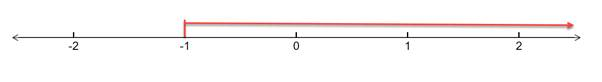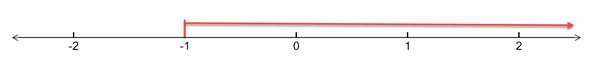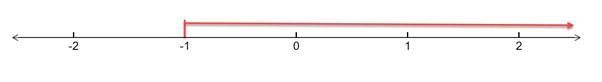# To solve the below inequality in terms of intervals and illustrate the solution set on the real number line - 1 − x ≤ 2### Single Variable Calculus: Concepts...

4th Edition
James Stewart
Publisher: Cengage Learning
ISBN: 9781337687805### Single Variable Calculus: Concepts...

4th Edition
James Stewart
Publisher: Cengage Learning
ISBN: 9781337687805

#### Solutions

Chapter A, Problem 13E
To determine

## To solve the below inequality in terms of intervals and illustrate the solution set on the real number line -   1−x≤2

Expert Solution

The solution of the inequality is x1 and the solution set on a real number line -### Explanation of Solution

Given: Inequality: 1x2

Formula Used:

An inequality compares two values, showing if one is less than, greater than, or simply not equal to another value.

Real number line is the line whose points are the real numbers.

Calculation:

Given: Inequality equation is 1x2

Solving the above equation, we have:

Subtract (1) from both the sides -

1x121

Solving further:

x1

Multiply both the sides by (1) and reversing the inequality, we have:

(x)×(1)(1)×(1)

x1

Drawing the above inequality on a real number line, we have:Conclusion:

Hence, the solution of the inequality is x1 and the solution set on a real number line -### Have a homework question?

Subscribe to bartleby learn! Ask subject matter experts 30 homework questions each month. Plus, you’ll have access to millions of step-by-step textbook answers!